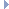# OpenGraph DrawingFramework

v. 2022.02 (Dogwood)

ogdf::Skeleton Class Referenceabstract

Skeleton graphs of nodes in an SPQR-tree. More...

#include <ogdf/decomposition/Skeleton.h>Inheritance diagram for ogdf::Skeleton:

## Public Member Functions

Skeleton (node vT)
Creates a skeleton S with owner tree T and corresponding node vT. More...

virtual ~Skeleton ()

GraphgetGraph ()
Returns a reference to the skeleton graph M. More...

const GraphgetGraph () const
Returns a reference to the skeleton graph M. More...

virtual bool isVirtual (edge e) const =0
Returns true iff e is a virtual edge. More...

virtual node original (node v) const =0
Returns the vertex in the original graph G that corresponds to v. More...

virtual const SPQRTreeowner () const =0
Returns the owner tree T. More...

virtual edge realEdge (edge e) const =0
Returns the real edge that corresponds to skeleton edge e. More...

edge referenceEdge () const
Returns the reference edge of S in M. More...

node treeNode () const
Returns the corresponding node in the owner tree T to which S belongs. More...

virtual edge twinEdge (edge e) const =0
Returns the twin edge of skeleton edge e. More...

virtual node twinTreeNode (edge e) const =0
Returns the tree node in T containing the twin edge of skeleton edge e. More...

## Protected Attributes

Graph m_M
actual skeleton graph More...

edge m_referenceEdge
reference edge More...

node m_treeNode
corresp. More...

## Detailed Description

Skeleton graphs of nodes in an SPQR-tree.

The class Skeleton maintains the skeleton S of a node vT in an SPQR-tree T. We call T the owner tree of S and vT the corresponding tree node. Let G be the original graph of T.

S consists of an undirected multi-graph M. If the owner tree of S is a PlanarSPQRTree, then M represents a combinatorial embedding. The vertices in M correspond to vertices in G. The edges in M are of two types: Real edges correspond to edges in G and virtual edges correspond to tree-edges in T having vT as an endpoint.

Let e in M be a virtual edge and eT be the corresponding tree-edge. Then there exists exactly one edge e' in another skeleton S' of T that corresponds to eT as well. We call e' the twin edge of e.

Definition at line 61 of file Skeleton.h.

## ◆ Skeleton()

 ogdf::Skeleton::Skeleton ( node vT )
inlineexplicit

Creates a skeleton S with owner tree T and corresponding node vT.

Precondition
vT is a node in T
Remarks
Skeletons are created by the constructor of SPQRTree and can be accessed with the skeleton() function of SPQRTree.

Definition at line 74 of file Skeleton.h.

## ◆ ~Skeleton()

 virtual ogdf::Skeleton::~Skeleton ( )
inlinevirtual

Definition at line 78 of file Skeleton.h.

## ◆ getGraph() [1/2]

 Graph& ogdf::Skeleton::getGraph ( )
inline

Returns a reference to the skeleton graph M.

Definition at line 104 of file Skeleton.h.

## ◆ getGraph() [2/2]

 const Graph& ogdf::Skeleton::getGraph ( ) const
inline

Returns a reference to the skeleton graph M.

Definition at line 99 of file Skeleton.h.

## ◆ isVirtual()

 virtual bool ogdf::Skeleton::isVirtual ( edge e ) const
pure virtual

Returns true iff e is a virtual edge.

Precondition
e is an edge in M

Implemented in ogdf::DynamicSkeleton, and ogdf::StaticSkeleton.

## ◆ original()

 virtual node ogdf::Skeleton::original ( node v ) const
pure virtual

Returns the vertex in the original graph G that corresponds to v.

Precondition
v is a node in M.

Implemented in ogdf::DynamicSkeleton, and ogdf::StaticSkeleton.

## ◆ owner()

 virtual const SPQRTree& ogdf::Skeleton::owner ( ) const
pure virtual

Returns the owner tree T.

Implemented in ogdf::DynamicSkeleton, and ogdf::StaticSkeleton.

## ◆ realEdge()

 virtual edge ogdf::Skeleton::realEdge ( edge e ) const
pure virtual

Returns the real edge that corresponds to skeleton edge e.

If e is virtual edge, then 0 is returned.

Precondition
e is an edge in M

Implemented in ogdf::StaticSkeleton, and ogdf::DynamicSkeleton.

## ◆ referenceEdge()

 edge ogdf::Skeleton::referenceEdge ( ) const
inline

Returns the reference edge of S in M.

The reference edge is a real edge if S is the skeleton of the root node of T, and a virtual edge otherwise.

Definition at line 94 of file Skeleton.h.

## ◆ treeNode()

 node ogdf::Skeleton::treeNode ( ) const
inline

Returns the corresponding node in the owner tree T to which S belongs.

Definition at line 85 of file Skeleton.h.

## ◆ twinEdge()

 virtual edge ogdf::Skeleton::twinEdge ( edge e ) const
pure virtual

Returns the twin edge of skeleton edge e.

If e is not a virtual edge, then 0 is returned.

Precondition
e is an edge in M

Implemented in ogdf::StaticSkeleton, and ogdf::DynamicSkeleton.

## ◆ twinTreeNode()

 virtual node ogdf::Skeleton::twinTreeNode ( edge e ) const
pure virtual

Returns the tree node in T containing the twin edge of skeleton edge e.

If e is not a virtual edge, then 0 is returned.

Precondition
e is an edge in M

Implemented in ogdf::StaticSkeleton, and ogdf::DynamicSkeleton.

## ◆ m_M

 Graph ogdf::Skeleton::m_M
protected

actual skeleton graph

Definition at line 146 of file Skeleton.h.

## ◆ m_referenceEdge

 edge ogdf::Skeleton::m_referenceEdge
protected

reference edge

Definition at line 145 of file Skeleton.h.

## ◆ m_treeNode

 node ogdf::Skeleton::m_treeNode
protected

corresp.

tree node in owner tree

Definition at line 144 of file Skeleton.h.

The documentation for this class was generated from the following file: